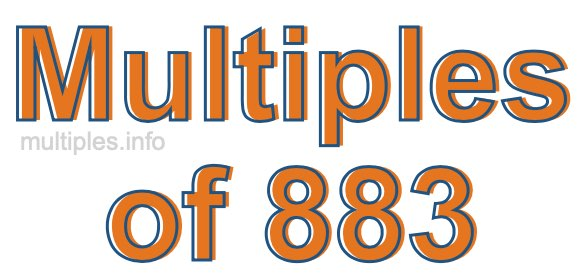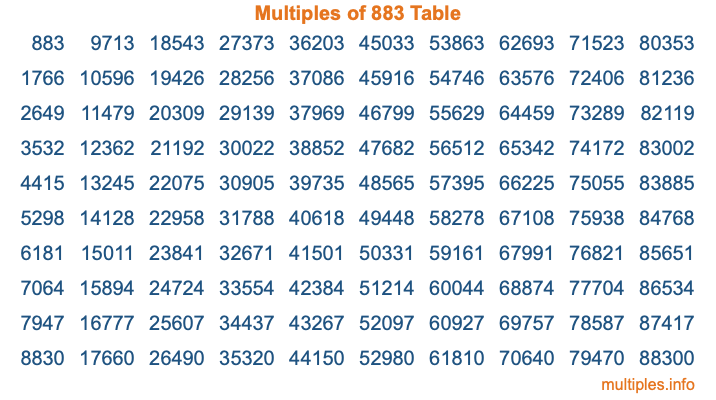Multiples of 883Welcome to the Multiples of 883 page. Here we will first teach you everything you will ever need to know about the multiples of 883, and then give you a study guide summary of everything we taught you to make sure you remember it all. Use this page to look up facts and learn information about the multiples of 883. This page will make you a multiples of eight hundred eighty-three expert!

Definition of Multiples of 883
Multiples of 883 are all the numbers that when divided by 883 equal an integer. Each of the multiples of 883 are called a multiple. A multiple of 883 is created by multiplying 883 by an integer.

Therefore, to create a list of multiples of 883, you start with 1 multiplied by 883, then 2 multiplied by 883, then 3 multiplied by 883, and so on for as long as you want. Thus, the list of the first five multiples of 883 is 883, 1766, 2649, 3532, and 4415. To see a larger list of multiples of 883, see the printable image of Multiples of 883 further down on this page. We also have a category where you can choose any nth multiple of 883.

Multiples of 883 Checker
The Multiples of 883 Checker below checks to see if any number of your choice is a multiple of 883. In other words, it checks to see if there is any number (integer) that when multiplied by 883 will equal your number. To do that, we divide your number by 883. If the the quotient is an integer, then your number is a multiple of 883.

Is  a multiple of 883?

Least Common Multiple of 883 and ...
A Least Common Multiple (LCM) is the lowest multiple that two or more numbers have in common. This is also called the smallest common multiple or lowest common multiple and is useful to know when you are adding our subtracting fractions. Enter one or more numbers below (883 is already entered) to find the LCM.

Check out our LCM Calculator if you need more details about the Least Common Multiple or if you need the LCM for different numbers for adding and subtraction fractions.

nth Multiple of 883
As we stated above, 883 is the first multiple of 883, 1766 is the second multiple of 883, 2649 is the third multiple of 883, and so on. Enter a number below to find the nth multiple of 883.

th multiple of 883

Multiples of 883 vs Factors of 883
883 is a multiple of 883 and a factor of 883, but that is where the similarities end. All postive multiples of 883 are 883 or greater than 883. All positive factors of 883 are 883 or less than 883.

Below is the beginning list of multiples of 883 and the factors of 883 so you can compare:

Multiples of 883: 883, 1766, 2649, 3532, 4415, etc.

Factors of 883: 1, 883

As you can see, the multiples of 883 are all the numbers that you can divide by 883 to get a whole number. The factors of 883, on the other hand, are all the whole numbers that you can multiply by another whole number to get 883.

It's also interesting to note that if a number (x) is a factor of 883, then 883 will also be a multiple of that number (x).

Multiples of 883 vs Divisors of 883
The divisors of 883 are all the integers that 883 can be divided by evenly. Below is a list of the divisors of 883.

Divisors of 883: 1, 883

The interesting thing to note here is that if you take any multiple of 883 and divide it by a divisor of 883, you will see that the quotient is an integer.

Multiples of 883 Table
Below is an image of the first 100 multiples of 883 in a table. The table is in chronological order, column by column. The first column has the first ten multiples of 883, the second column has the next ten multiples of 883, and so on.The Multiples of 883 Table is also referred to as the 883 Times Table or Times Table of 883. You are welcome to print out our table for your studies.

Negative Multiples of 883
Although not often discussed or needed in math, it is worth mentioning that you can make a list of negative multiples of 883 by multiplying 883 by -1, then by -2, then by -3, and so on, to get the following list of negative multiples of 883:

-883, -1766, -2649, -3532, -4415, etc.

Multiples of 883 Summary
Below is a summary of important Multiples of 883 facts that we have discussed on this page. To retain the knowledge on this page, we recommend that you read through the summary and explain to yourself or a study partner why they hold true.

There are an infinite number of multiples of 883.

A multiple of 883 divided by 883 will equal a whole number.

883 divided by a factor of 883 equals a divisor of 883.

The nth multiple of 883 is n times 883.

The largest factor of 883 is equal to the first positive multiple of 883.

883 is a multiple of every factor of 883.

883 is a multiple of 883.

A multiple of 883 divided by a divisor of 883 equals an integer.

883 divided by a divisor of 883 equals a factor of 883.

Any integer times 883 will equal a multiple of 883.

Multiples of a Number
Here you can get the multiples of another number, all with the same attention to detail as we did for multiples of 883 on this page.

Multiples of
Multiples of 884
Did you find our page about multiples of eight hundred eighty-three educational? Do you want more knowledge? Check out the multiples of the next number on our list!